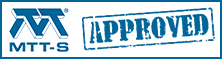# Transmission Line Loss

Here we will review some of the math for calculating the RF losses (attenuation) of transmission lines over frequency. We'll guide you to some of our other pages that show calculations of attenuation of different transmission line geometries.

Transmission line loss Rule of Thumb 1

Attenuation or rejection?

Transmission line model

Four loss mechanisms:

Transmission line rule of thumb 2

Attenuation due to metal (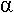C)

Attenuation due to dielectric loss tangent (D)

Attenuation due to dielectric conductivity (G)

Attenuation due to radiation (R)

Let's propose a rule of thumb before we even get started discussing transmission line losses: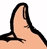Transmission line attenuation Rule of Thumb #80

For a given frequency, waveguide will give the lowest loss per unit length. Coax loss will be about 10X higher (in dB). Transmission line loss on MMICs (microstrip or coplanar waveguide) is about 10X worse than coax, or 100X that of waveguide (but the lengths of the transmission lines are really small!) Stripline, depending on its geometry, usually will be slightly higher in loss than coax.

### Attenuation or rejection?

We need to draw a distinction between "real" loss (attenuation) and mismatch loss (rejection). A filter can be used to reject a signal, but rejection usually means reflection. The rejected signal is returned to the generator where it is dissipated. With an appropriate matching network, the "loss" of a filter could be theoretically tuned out to zero dB.

Attenuation can be reduced by increasing system characteristic impedance (usually not an option), but cannot be completely tuned away, unless you are able to change the characteristic impedance to infinity. This is never an option!

### Transmission line model

The transmission line model is used in many of the loss calculations. When you solve Maxwell's Equations for electromagnetic wave propagation, the electric field solutions look like this: E(z,t) = E * cos(γz - ωt).
Setting the argument of the cos function to a constant is like picking a point on the wave and riding on it.
Solving for z we get:

z=(ω/γ)t.

Let γ =α + jβ or α + j(2π/λ)

Now we have arrived at the individual properties that define the circuit performance and that are commonly used in our transmission line solutions, where:

γ = complex propagation constant
α = attenuation constant (nepers/unit length)
β = phase constant (radians/unit length)
λ = wavelength

We recommend that you study our page on propagation constant, and in particular become familiar with the "attenuation constant" before you continue reading this page on transmission line loss. The propagation constant is what determines the phase and amplitude of a signal on a transmission line. It is denoted by Greek letter gamma:

The transmission line model is for an infinitesimal section of line, the line can be composed of four lumped elements: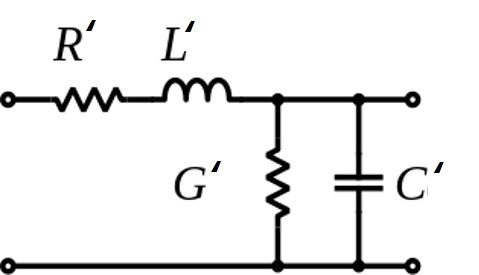Note that the "prime" notation here means that parameters are normalized to length. C' is in Farads/meter, L' is in Henries/meter, R' is in Ohms/meter, and G' is in Siemens/meter. A wealth of transmission line parameters can be expressed in terms of of these four lumped elements, including characteristic impedance, propagation constant and phase velocity.

### Four types of losses

To quantize the RF losses in transmission lines we need to calculate the attenuation constant, which is in the "natural" units of Nepers/meter. The attenuation constant can be broken down into at least four components, one representing metal loss, one representing dielectric loss due to loss tangent, one due to conductivity of the dielectric, and one due to stray radiation: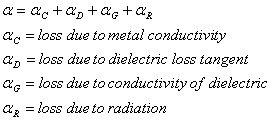Below we'll deal with each of these loss mechanisms in terms of the transmission line model.

Each of the four components of loss are geometry-dependent, meaning the calculation is quite different for coax than it is for waveguide for example. We'll just touch on each subject below, and move most of the math to separate pages on different transmission lines. But first it's time for another Microwaves101 Rule of Thumb:

#### Transmission line attenuation Rule of Thumb #79Different loss mechanisms have different behaviors over frequency. Metal loss is proportional to square-root frequency. Dielectric loss is proportion to frequency. Dielectric conduction loss is constant over frequency.

#### Attenuation due to metal conductivity (C)

The overall loss of most transmission lines is dominated by the metal loss at microwave frequencies. Metal loss varies predominantly as SQRT(f). Metal loss is modeled by the R' component in the transmission line model which is series resistance per unit length. The R' term is a function of the geometry of the transmission line and the RF sheet resistance of the metal system that is used.

The RF sheet resistance can be far more than the DC sheet resistance (thanks, Loren!), because of the skin effect. The skin effect says that at higher and higher frequency, the path of the signal bunches up toward the outside of a conductor, on the surface where the EM wave is propagating.

Metal loss varies predominantly as SQRT(f) because RF sheet resistance varies as SQRT(f). The RF sheet resistance for a single-metal system is calculated as: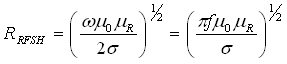Sigma is the metal's conductivity in Siemens/meter; sigma is 1/rho, where rho is resistivity and is in units of Ohm-meters. RF sheet resistance units are Ohms/square. The calculation for RF sheet resistance of multiple-layer metal systems is explained on our skin depth page. Here's the RF sheet resistance versus frequency for three mils of copper (many, many skin depths at microwave frequencies), which you can regard as the best you'll ever do, unless someone starts making printed wiring boards with pure silver metal traces, silver has lower bulk resistivity than copper.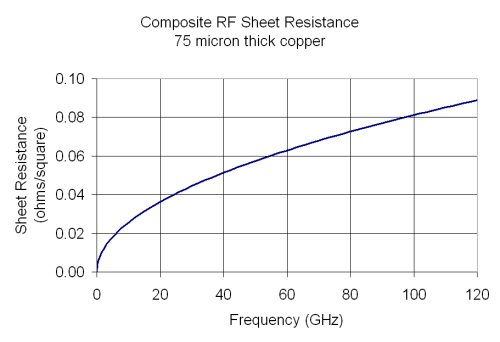The next step in calculating transmission line metal loss is to find the RF resistance per unit length R' (convert Ohms/square to Ohms/meter), based on the physical dimensions of the structure and the RF sheet resistance. This calculation is different for coax, waveguide, microstrip or any other transmission line structure. The devil is in the details! The links below will help you with this part of the calculation. Note that with the exception of waveguide, resistance per length has two components in transmission lines; you have to sum up the contribution of the "hot" conductor with the ground plane conductor (or ground plane conductors in the case of coplanar waveguide).

The final step in calculating RF loss due to metal: from the resistance per unit length, you find the loss per unit length: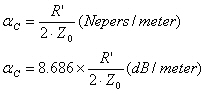Notice the "natural" units of transmission line loss are Nepers/length. To get loss in dB/length, multiply Nepers by 8.686.

Click here to go to our page on microstrip loss due to metal

Click here to go to our page on coax loss due to metal

Click here to go to our page on waveguide loss due to metal

Click here to go to our page on minimizing attenuation due to metal

#### Attenuation due to dielectric loss tangent (D)

Loss due to dielectric loss tangent (tanδ) can be very important at microwave frequencies. Loss tangent is also known as dissipation factor, or "DF" as an abbreviation. This term is proportional to frequency, so the higher you go, the more likely it will dominate overall loss (metal loss is proportional to SQRT of frequency).

The calculation for loss due to loss tangent is straightforward and uses the elements of the transmission line model with one stipulation. Did we mention that the capacitor C' in the transmission line might be lossy? The loss tangent is a measure of the ratio of its conductance to its susceptance (like "Q"). Once you have calculated capacitive susceptance/length for the specific geometry, all you need to do is multiply by the loss tangent to get the frequency-dependent conductance term that causes loss tangent loss: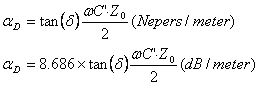For any TEM transmission line of any impedance, the equation for dielectric loss tangent loss can be simplified to the following: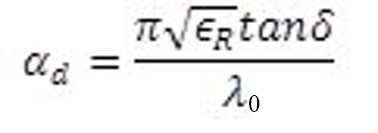(Nepers/length)

Here the length units will be consistent with units you use for wavelength. Making the conversion from Nepers to dB (multiplying by 8.686) results in a more familiar result: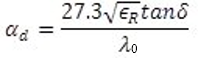(dB/length)

Let's go one step farther than most textbooks. Who spends a lot of time thinking about free space wavelength? Here is the equation in terms of frequency: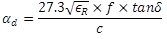(dB/length)

And further, let's assume frequency is in GHz, and speed of light is 29.979 cm/nanosecond. Then: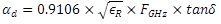(dB/cm)

Notice that loss tangent loss is independent of geometry. Although you might reduce metal loss by going to fatter coax, you will still suffer the same dielectric loss if you are using the same dielectric material.

At the risk of repeating ourselves, loss tangent loss is proportional to frequency, as opposed to metal loss which is proportional to SQRT(frequency). What this means is, as you go higher in frequency, loss tangent starts to dominate the loss of a transmission line at some point. If you are working up at W-band, you can no longer go by the advice of your X-band predecessors which might have been "just ignore it". Further, it becomes more important than ever to obtain accurate loss tangent data. If you scour the web you will see widely-different values for loss tangent of mature substrate technology such as GaAs. This is an industry-wide problem, and maybe a good career to consider (material measurements). Note that loss tangent is often a function of frequency, temperature, material quality.... so don't ever hang your hat on a single number.

Now let's look at a plot of loss tangent loss. Here we swept tanδ from 0 to 0.01 in increments of 0.001, for Er=1. Check it out, loss is almost exactly 1 dB/cm at 110 GHz for 1% loss tangent (for the impossible condition that Er=1). If you can remember that one data point you could scale loss tangent loss in your head, if you didn't have such a hangover. But don't forget to multiply by SQRT(Er)!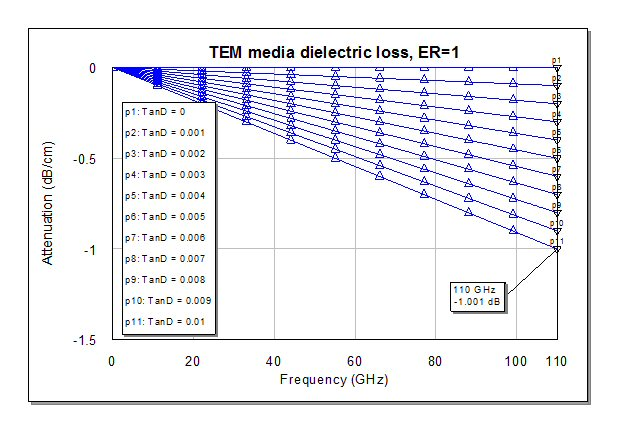Speaking of Er, what happens if you are using a quasi-TEM transmission line, like CPW or microstrip? It turns out that you can substitute Keff for Er in the above equation and get very nearly the correct result (go ahead and verify this using EDA software if you don't trust us). Here's an example: consider microstrip-on-GaAs with loss tangent=0.0016 and Er=12.9. Ignoring (for the moment) the Er component, loss at 110 GHz would be 0.16 dB/cm. An educated guess for Keff in any microstrip line is that 70% of the fields are contained in the substrate, so Keff is ~9. Take the square root (3) and multiply 0.16 dB/cm you will estimate that loss tangent loss on a GaAs MMIC ~0.5 dB/cm. Note that metal loss will be the dominant effect at most frequencies of interest....

Now it's time for another Microwaves101 Rule of Thumb:

#### Loss tangent loss Rule of Thumb #116TEM transmission line, loss tangent of 0.01 (which is pretty high) results in almost exactly 1 dB/cm loss at 110 GHz, before you scale it by SQRT(dielectric constant). Since it is linear with frequency, you should be able to scale loss tangent attenuation in your head. You can approximate attenuation in microstrip or CPW if you scale by the effective dielectric constant.

Click here to go to our page on coax loss due to dielectric loss tangent

#### Attenuation due to substrate conductivity (G)

Note: many textbooks link substrate conduction loss term in with the loss tangent loss term. We choose to separate them, they behave quite differently.

Owing to the great dielectrics we have available for coax (such as PTFE) and MMICs (GaAs), the loss due to substrate conductivity term is often ignored because it's usually very small because the dielectric has extremely low conductivity. However, the proliferation of silicon into the microwave realm has brought this term back to our attention, because silicon has relatively poor electrical insulating properties (a true semiconductor!) Let's add another Microwaves101 Rule of Thumb to draw the line where substrate conductivity needs to be considered:

#### Transmission line loss Rule of Thumb #80When considering the loss of a transmission line due to dielectric conductivity, if the resistivity of the dielectric is greater than 10,000 Ohm-cm, forget it! That pretty much rules out all substrates except silicon, which can be anywhere from 1 Ohm-cm (very lossy) to 10,000 Ohm-cm (very expensive float-zone silicon). PTFE is 1E18 Ohm cm!

Here's the generic equation for this loss mechanism using the G' element of the transmission line model: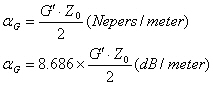One thing you should know about loss due to substrate conductivity: it's NOT a function of frequency! So if you use a cheap Ohmmeter to measure DC resistance across a transmission line's two conductors (and don't terminate the other end, that would be stupid!), you have what you need to predict loss due to conduction at 10 GHz!

Another thing you should know: for transmission line media with uniform dielectric media (coax, stripline) the calculation of G' is essentially the same calculation as C', except you substitute the dielectric's conductivity in place of its permittivity. We'll go into this in further detail on the page links below.

Click here to go to our page on microstrip loss due to substrate conductivity

Click here to go to our page on coax loss due to dielectric conductivity

#### Attenuation due to radiation (R)

This is another attenuation mechanism that has a very small effect, if your circuits are behaving well. It really isn't attenuation in the sense of the word that the energy goes up in heat, it is more of a leakage loss. But the effect upon your signal is the same either way, it loses energy.

In general, thicker substrates give up more radiation loss than thinner ones, and bends will radiate more than straight sections of lines. You can avoid radiation loss if you stick to waveguide, and minimize it if you use stripline.

There's no easy way to account for attenuation due to radiation using the transmission line model or with the popular linear simulators such as Microwave Office or ADS. That's one reason why 3D electromagnetic structure simulators are so popular!

New for December 2015: here's some free MatLab code that will help you calculate radiation loss, thanks to Sven van Berkel on our message board!  It is based on a quasi-analytical approach and was written at TU Delft.  Check it out and tell us how it works for you. It's a stand-alone executable file so you don't need a MatLab license. All the libraries needed are included in the executable. Thanks!

http://terahertz.tudelft.nl/Research/project.php?id=74&ti=27

Author : Unknown Editor